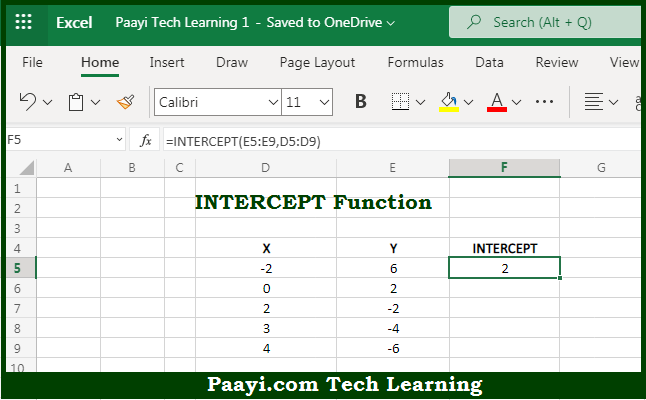# Learn How to Use Microsoft Excel INTERCEPT Function

Written by | 0 Comments | 607 Views

In this article, you will learn how to use the Microsoft Excel INTERCEPT function and its prime function in Microsoft Excel. You will also get to know the Microsoft Excel INTERCEPT function return value and syntax with the help of some examples.

Microsoft Excel INTERCEPT Function

The main purpose of the Microsoft Excel INTERCEPT function is to get the intercept of a linear regression line. That implies, with the help of the INTERCEPT function you can able to return the point at which a regression line will intersect the y-axis based on known x and y values. So, with the help of the INTERCEPT function, you can able to get the intercept of the linear regression line.

Return Value of INTERCEPT Function

The return value will be the y-axis intercept value.

Syntax of INTERCEPT Function

=INTERCEPT(known-ys, known-xs)

Where the arguments:

• known-ys: This is the array or range of numeric data points which are dependent values.
• known-xs: This is the array or range of numeric data points, which are independent values (optional).

## How to Use Microsoft Excel INTERCEPT Function?So we know that Microsoft Excel INTERCEPT function you can able to get the intercept of a linear regression line. That implies, with the help of the INTERCEPT function you can able to return the point at which a regression line will intersect the y-axis based on known x and y values. So, with the help of the INTERCEPT function, you can able to get the intercept of the linear regression line.